Math resources Number and quantity

Factors and multiples

# Factors and multiples

Here we will learn about factors and multiples, including their definitions, finding factors and multiples, and problem solving with factors and multiples.

Students will first learn about factors and multiples as part of their work with operations and algebraic thinking in elementary school.

## What are factors and multiples?

Factors are numbers that multiply together to find a product. They will divide into a whole number with no remainder and can sometimes be called divisors.

Composite numbers are numbers with more than two factors. Prime numbers are numbers with exactly two factors.

Multiples are the products you get when two factors, or numbers, are multiplied together.

Multiples are the result of multiplying a number by an integer.

Let’s look at factors and multiples.

FactorsMultiples

What are the factors of 8?

8 \times 1 = 8

1 and 8 are a factor pair.

2 and 4 are a factor pair.

You can use a multiplication chart
to find factors. The first row and
first column on the chart represent
the factors.

8 is a composite number because
it has more than two factors.

● Whole numbers have a set number
or a finite number of factors.

What are the multiples of 8?

8 \times 1 = 8

Some of the multiples of 8:

8, 16, 24, …

You can use a multiplication chart
see some of the multiples of 8. You
can read down the 8’ s column or
read across the 8 ’s row.

● Whole numbers have many or an
infinite number of multiples.

### What are factors and multiples?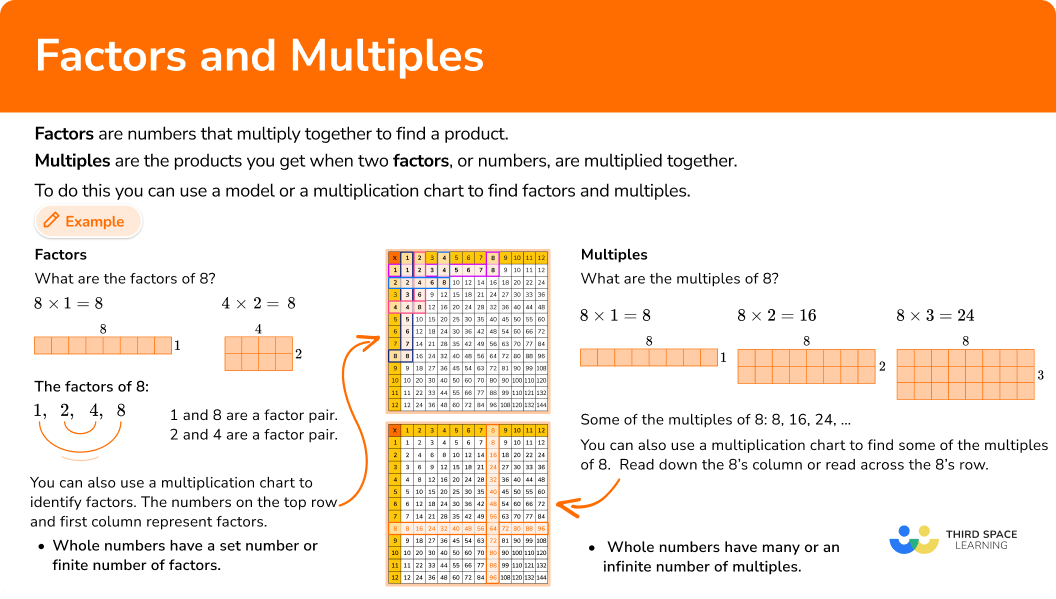## Common Core State Standards

How does this relate to 3rd, 4th, and 6th grade math?

• Grade 3: Operations & Algebraic Thinking (3.OA.B.5)
Apply properties of operations as strategies to multiply and divide.

• Grade 4: Operations & Algebraic Thinking (4.OA.B.4)
Find all factor pairs for a whole number in the range 1–100. Recognize that a whole number is a multiple of each of its factors. Determine whether a given whole number in the range 1–100 is a multiple of a given one-digit number. Determine whether a given whole number in the range 1–100 is prime or composite.

• Grade 6: Number System (6.NS.B)
Compute fluently with multi-digit numbers and find common factors and multiples.

## How to list factors and multiples

In order to list all of the factors of a given number:

1. State the factor pair starting with 1 \; \textbf{×} \; the number.
2. Write the next smallest factor of the number and calculate its factor pair.
3. Repeat until the next factor pair is the same as the previous pair.
4. Write out the list of factors for a number.

In order to find the common factors of a set of given numbers.

1. State the factor pair starting with 1 \; \textbf{×} \; the number for the set of numbers.
2. Write the next smallest factor of the number and calculate its factor pair for the set of numbers.
3. Repeat until the next factor pair is the same as the previous pair.
4. Write out the list of common factors for the set of numbers.

In order to find the multiples of a number.

1. State the first multiple of the number starting with 1 \; \textbf{×} \; that number.
2. Calculate the second multiple of the number.
3. Continue until you have calculated the number of multiples needed.
4. List the multiples.

In order to find at least one common multiple of a number.

1. Use the multiplication chart to find the rows or columns of each of the numbers.
2. Read across the rows or down the columns to find at least one set of matching multiples.

## Factors and Multiples examples

### Example 1: list the factors (odd number)

List the factors of 15.

1. State the factor pair starting with 1 \; \textbf{×} \; the number.

The factor pair is 1\times{15}.

2Write the next smallest factor of the number and calculate its factor pair.

15 is an odd number so 2 is not a factor of 15.

However, 15\div{3}=5 and so the next factor pair is 3 \times 5

3Repeat until the next factor pair is the same as the previous pair.

So far we have:

\begin{aligned} &1\times{15} \\ &3\times{5} \\ \end{aligned}

15 has a remainder when it is divided by 4, 6, 7, 8, 9.

The next factor to try is 5, but we already know that 3 \times 5 is a factor pair and this is the same as 5 \times 3.

We have now found all of the factor pairs:

\begin{aligned} &1\times{15}\\ &3\times{5} \end{aligned}

4Write out the list of factors for a number.

Reading down the first column of factors, and up the second column, the factors of 15 are 1, 3, 5, and 15.

### Example 2: listing factors (even number)

List the factors of 18.

The factor pair is 1 \times 18.

18 is an even number so 2 is a factor of 18.

18\div{2}=9 and so the next factor pair is 2 \times 9

So far we have:

\begin{aligned} &1\times{18}\\ &2\times{9}\\ \end{aligned}

18 does not have a remainder when it is divided by 3.

The next factor is 3, \; 3 \times 6 is the factor pair.

18 has a remainder when it is divided by 4

The next factor to try is 6, but we already know that 3 \times 6 is a factor pair and this is the same as 6 \times 3.

18 has a remainder when it is divided by 7, 8

The next factor to try is 9 but we already know that 2 \times 9 is a factor pair and this is the same as 9 \times 2

We have now found all of the factor pairs:

\begin{aligned} &1\times{18}\\ &2\times{9}\\ &3\times{6}\\ \end{aligned}

Reading down the first column of factors, and up the second column, the factors of 18 are 1, 2, 3, 6, 9, and 18.

### Example 3: finding the common factors

Find the common factors of 21 and 6.

The first factor pair for 21 is 1 \times 21

The first factor pair for 6 is 1 \times 6

21 is an odd number so 2 is not a factor of 21.

However, 21\div{3}=7 and so the next factor pair of 21 is 3 \times 7

6 is an even number so 2 is a factor of 6.

6\div{2}=3 and so the next factor pair of 6 is 2 \times 3

So far for 21, we have:

\begin{aligned} &1\times{21}\\ &3\times{7}\\ \end{aligned}

21 has a remainder when it is divided by 4, 5, 6, 8, 9.

The next factor to try is 7, but we already know that 3 \times 7 is a factor pair, and this is the same as 7 \times 3.

We have now found all of the factor pairs:

\begin{aligned} &1\times{21}\\ &3\times{7}\\ \end{aligned}

So far for 6 we have:

\begin{aligned} &1\times{6}\\ &2\times{3}\\ \end{aligned}

6 does not have a remainder when it is divided by 3. The next factor of 6 is 3 but we already know that 2 \times 3 is a factor pair and this is the same as 3 \times 2.

The factors of 21 are 1, 3, 7, 21

The factors of 6 are 1, 2, 3, 6.

1 and 3 are the common factors of 6 and 21.

### Example 4: listing multiples

List the first five multiples of 7

7\times{1}=7

The second multiple of 7 is

7\times{2}=14.

7\times{3}=21

7\times{4}=28

7\times{5}=35

The first 5 multiples of 7 are 7, 14, 21, 28, and 35.

Reading across the 7’s row or down the 7’s column on a multiplication chart, you can see that those multiples are correct.

### Example 5: listing multiples (two digit number)

List the first 5 multiples of 12.

The first multiple of 12 is

12\times{1}=12.

The second multiple of 12 is

12\times{2}=24.

12\times{3}=36

12\times{4}=48

12\times{5}=60

The first 5 multiples of 12 are 12, 24, 36, 48, and 60.

Reading across the 5’s row or down the 5’s columns on a multiplication chart, you can see that those multiples are correct.

### Example 6: common multiples

Find one common multiple of 5 and 9.

5\times{9}=45

9\times{5}=45

45 is a common multiple of 5 and 9.

### Teaching tips for multiples

• To help students see the patterns in the numbers, use a multiplication table so that students can see examples of multiples and look for patterns.

• Instead of using printable math worksheets, have young learners play games to practice their multiplication facts and remember divisibility rules. There are many digital multiples games and factors games at the fourth grade level to help students.

• Identifying factors is usually more difficult for students than finding multiples. Using manipulatives, such as counters, and games help struggling learners find positive factors.

### Easy mistakes to make

• Confusing multiples and factors
Factors and multiples often get mixed up. Factors of a number are the whole numbers that divide into it with no remainder. Multiples are numbers that you get when you multiply one whole number by another whole number.

• Forgetting that 1 and the number itself represent a factor pair.
All numbers are a factor of themselves and 1 is a factor of every number.
For example, the factors of 6 are 1, 2, 3, and 6, so 6 is a factor of itself.

• Remember the number itself for multiples
All numbers are a multiple of themselves.
For example, the multiples of 6 are 6, 12, 18, 24 and so on, so 6 is a multiple of itself.

### Practice factors and multiples questions

1. List the factors of 24.

24, 48, 72, 9612, 24, 36, 481, 2, 3, 4, 6, 8, 12, 242, 3, 4, 6, 8, 12The first factor of 24 is 1.

1\times{24} is the first factor pair

The next factor of 24 is 2 since 24 is an even number.

2\times{12} is the next factor pair.

3, 4, 6, and 8 divide into 24 without remainders. So, the next factor pairs are

\begin{aligned} &3\times{8}\\ &4\times{6} \end{aligned}

So the factors of 24 are 1, 2, 3, 4, 6, 8, 12, and 24.

2. List the factors of 15.

1, 3, 5, 153, 515, 30, 45, 605, 15, 30, 45The first factor of 15 is 1.

1\times{15} is the first factor pair.

15 is not an even number so 2 is not a factor. 3 and 5 divide into 15 without a remainder.

So the next factor pair is: 3\times{5}

3\times{5} and 5\times{3} are the same factor pair.

So the factors of 15 are 1, 3, 5, and 15.

3. Find the common factors of 8 and 20.

2, 440, 1601, 81, 2, 4List the factors of 8 and 20.

The first factor of 8 is 1.

1\times{8} is the first factor pair.

8 is an even number so 2 will be the next factor of 8.

2\times{4} is the next factor pair.

3, 5, 6, 7, 9 are not factors of 8 because they do not divide into 8 without a remainder.

The first factor of 20 is 1.

1\times{20} is the first factor pair.

20 is an even number, so 2 divides 20 without a remainder.

2\times{10} is the next factor pair.

3 is not a factor of 20. \; 4 and 5 are factors of 20.

4\times{5} is the next factor pair. 5\times{4} is the same factor pair as 4\times{5}.

6, 7, 8, 9 are not factors of 20 because they do not divide 20 without a remainder.

The factors of 8 are 1, 2, 4, 8

The factors of 20 are 1, 2, 4, 5, 10, 20

The common factors are 1, 2, 4

4. List the first 5 multiples of 6.

6, 12, 18, 24, 301, 2, 3, 5, 612, 18, 24, 30, 366, 12, 24, 36, 42List the first multiple of 6 which is 1\times{6}=6

List the second multiple of 6 which is 2\times{6}=12

Continue this pattern until you have 5 multiples.

3\times{6}=18

4\times{6}=24

5\times{6}=30

The first 5 multiples of 6 are: 6, 12, 18, 24, 30

Using the multiplication chart you can see that the first 5 multiples are 6, 12, 18, 24, 30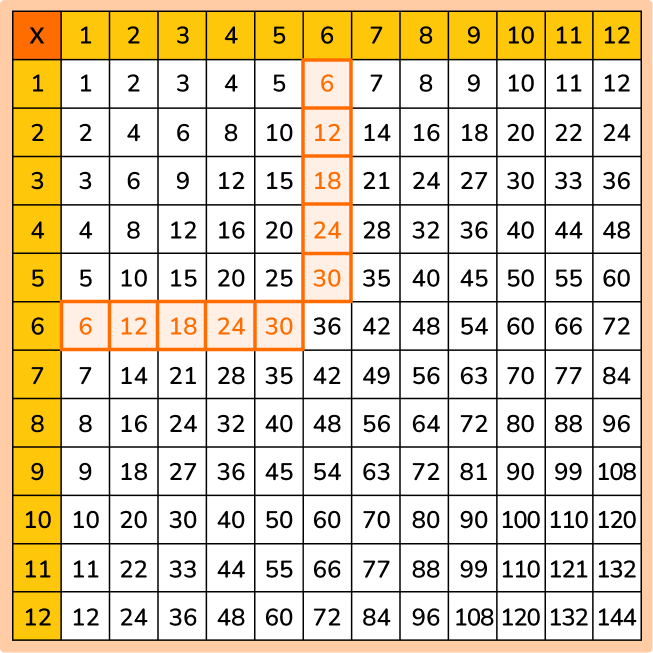5. List the first 5 multiples of 10.

1, 2, 5, 10, 2010, 20, 30, 40, 5020, 30, 40, 50, 602, 5, 10, 20, 25List the first multiple of 10 which is 1\times{10}=10

List the second multiple of 10 which is 2\times{10}=20

Continue this pattern until you have 5 multiples.

3\times{10}=30

4\times{10}=40

5\times{10}=50

The first 5 multiples of 10 are: 10, 20, 30, 40, 50

Using the multiplication chart you can see that the first 5 multiples are 10, 20, 30, 40, 50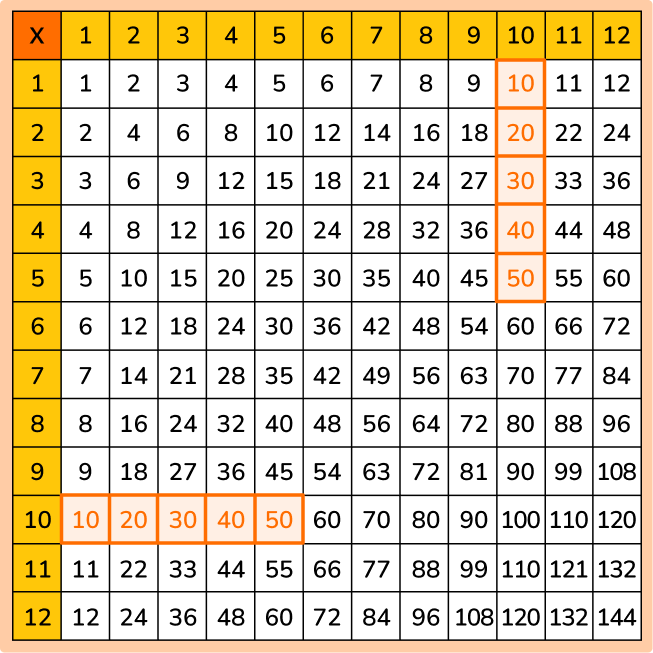6. Find one common multiple of 5 and 7.

12253530Use the multiplication chart by looking at all the multiples in the 5’s row and all the multiples in the 7’s row.

Find a pair of multiples that match.

35 is the matching multiple. 35 is one common multiple of 5 and 7.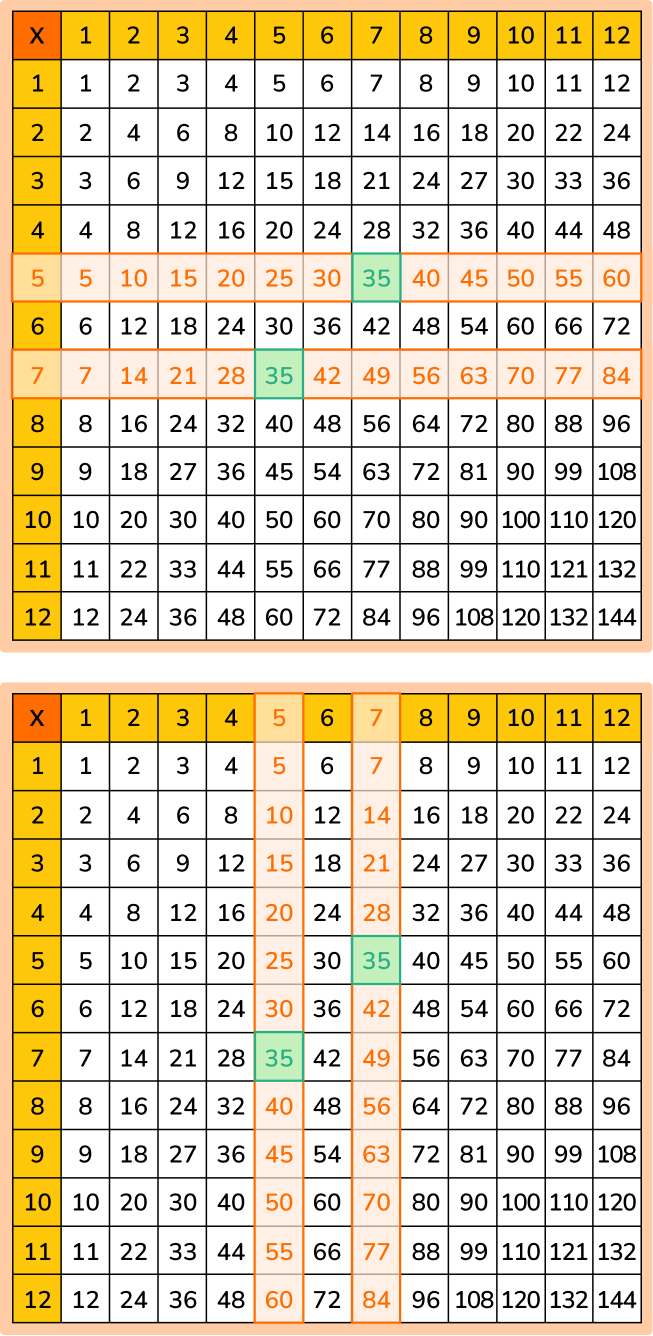## Factors and Multiples FAQ

Are factors and multiples the same thing?

No, factors and multiples are not the same. Factors are two or more whole numbers multiplied together that give a product. In other words, a factor divides a number and leaves no remainder. A multiple is the product when one number is multiplied by another number.

Are factors and multiples always positive numbers?

No, factors and multiples can be negative numbers. However, in elementary school, students typically work only with positive numbers.

Do prime numbers have factors and multiples?

Prime numbers have an infinite number of multiples but only 2 factors.

Are there other strategies to find factors and multiples besides using multiplication charts?

Yes, you can use a number line to find factors and multiples and to skip count.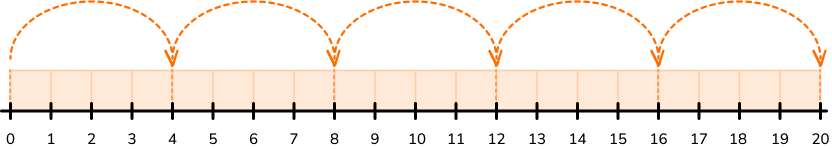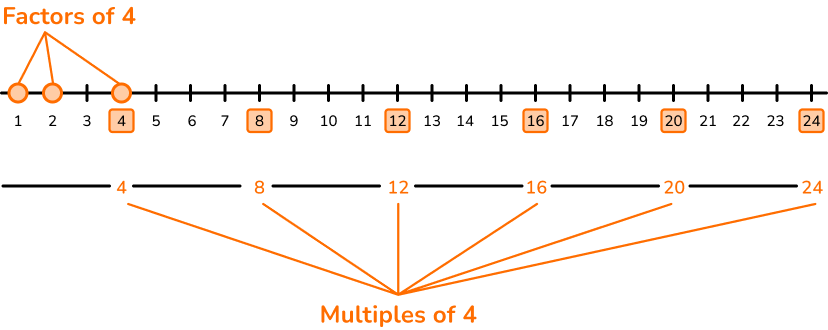## Still stuck?

At Third Space Learning, we specialize in helping teachers and school leaders to provide personalized math support for more of their students through high-quality, online one-on-one math tutoring delivered by subject experts.

Each week, our tutors support thousands of students who are at risk of not meeting their grade-level expectations, and help accelerate their progress and boost their confidence.